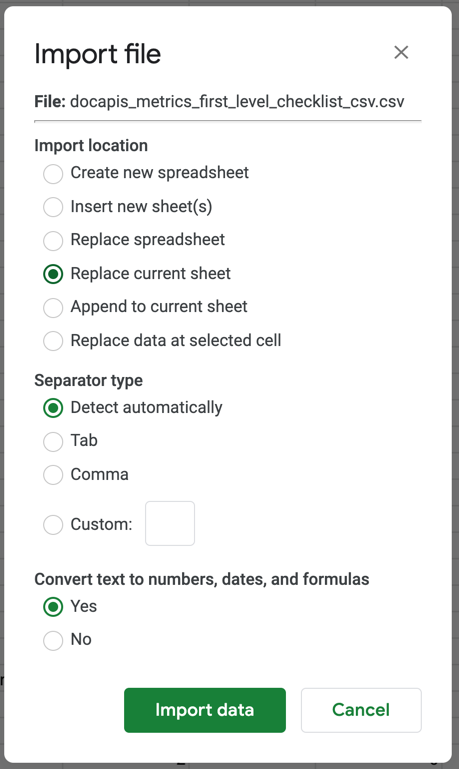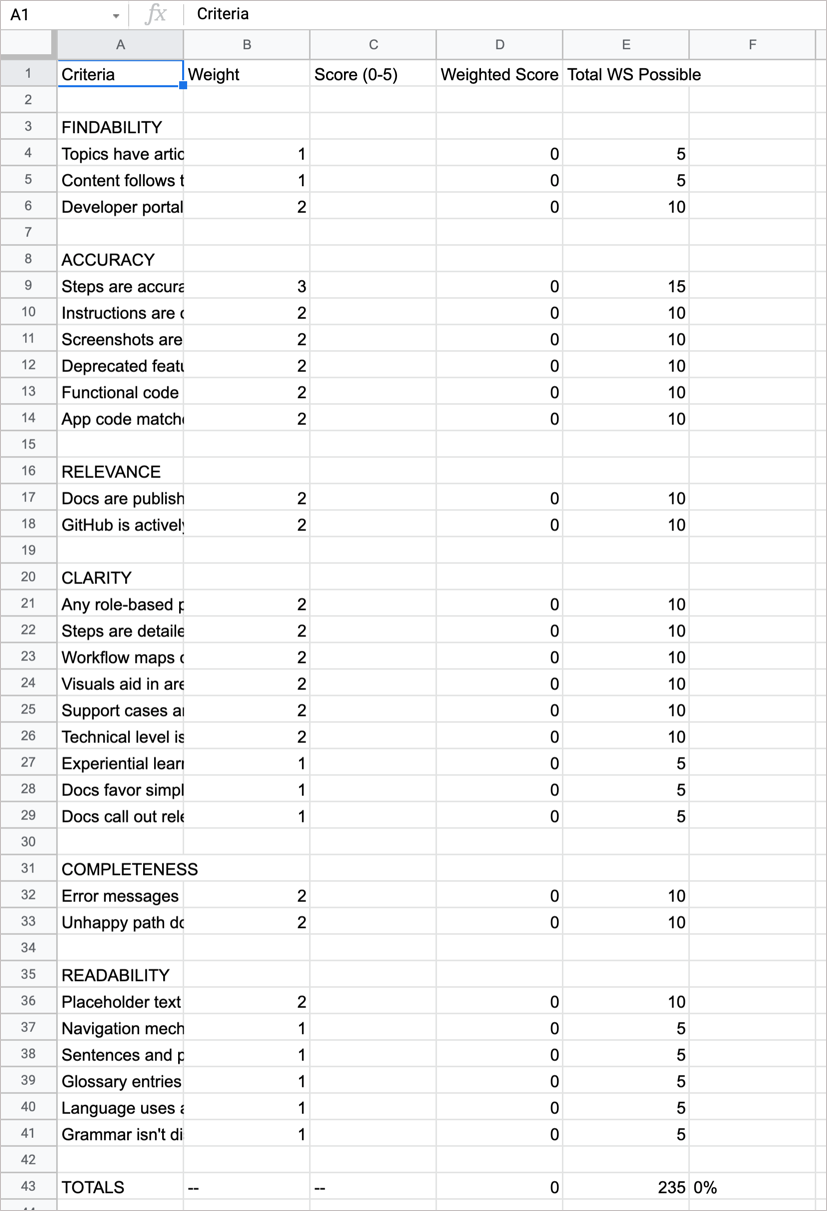Templates for working with metrics

Last updated: Feb 15, 2021

You can use two templates for the metrics described in this Metrics and measurement section. These templates were designed to work with Google Docs / Microsoft Word and Google Sheets / Microsoft Excel. The purpose of the doc template is for narrative/qualitative assessments, while the spreadsheet template is to more easily calculate the scores.

Using the doc templates for narrative assessments

The purpose is the doc templates is make it easier to do narrative assessments of the various criteria. For example, if you find that the docs lack tutorials for the key use cases of the API, make notes about what use cases are lacking, or maybe note your inability to locate certain use cases in the docs. These kinds of narrative assessments don’t work well in spreadsheets. Here are the two doc templates:

The templates strip away most formatting and just list the various criteria in a basic HTML file. Copy and paste the content into Google Docs or Microsoft Word. Then as you go through the content, make your notes in the “Assessment” area. Also choose a score of 0 to 5. (Weighting for each criteria is done in the spreadsheet, not in the doc template.)

The spreadsheets are meant to facilitate scoring. It’s not possible to do a lot of calculations in a Google Docs or Microsoft Word, so you’ll need to copy over each score from the doc template into the right cells into a spreadsheet. The CSV file imports equally into Google Sheets or Microsoft Excel, but the steps are a bit different.

To use the spreadsheet to calculate a score:

1. Right-click the links below and select Save Link As (or similar for other browsers) to save the files to your computer:

2. If you’re using Microsoft Excel (the app, not the browser version), open the CSV file in Microsoft Excel and save the file as an XSLS file. Then skip ahead to step 4.
2. In the new spreadsheet, go to File > Import. In the Import file window, click the Upload tab.
3. Drag the CSV file you downloaded in step 1 into the “Drag a file here” box.
4. In the Import File dialog box that appears, under Import Location, select Replace current sheet. Leave the other default options as is. (Specifically, make sure “Convert text to numbers, dates, and formulas” is set to Yes because the CSV file has formulas.)4. After importing, get familiar with the spreadsheet columns.The spreadsheet looks plain, but there are some formulas that do calculations here. You can add formatting if you want (for example, bold the top row, expand column A), or change the weighting, but don’t add or remove the columns or rows. If you change any of the rows or columns, the formulas will no longer work because they reference specific cells in specific columns.

• Criteria: Shows the title (but not description) of each criteria.
• Weight: Indicates how much weight the criteria should be given. Feel free to change this as you see fit. When you update the weight, the Total WS Possible changes (because it just multiples 5 x the weight).
• Score: This is where you enter your score. It’s the only column you work with. Copy this number from your Google Docs spreadsheet to here.
• Weighted Score: Has formulas to calculate the weight times the score. This is automatically calculated when you input a score. The formulas for these cells appear if you click in a cell. For example, =(B4*C4), where 4 changes for each row. You can adjust the weight for each criteria as desired. Currently, the weight is 1 to 3 points.
• Total WS Possible: The total weighted score possible. If the score were perfect for each criteria (5), this would be the maximum weighted score. For example, if the weight is 3 and the score is 5, the total weighted score possible would be 15. This field is automatically calculated based on the weight. If you change the weight, this field updates. For example, the formula is =(B4*5), where 4 changes for each row.

At the bottom is a row called “TOTALS.” This row gets the sum of the Weighted Score, the sum of the Total WS Possible, and then divides the two to arrive at a total score.

For example, suppose the total Weighted Score was 120, and the Total Weighted Score Possible was 235. Then the total score would be 120/235, or 51%.

The formula driving the calculation of the percent is as follows: =ROUND(((D61/E61)*100),0)&"%" (for the first-level checklist), and =ROUND(((D43/E43)*100),0)&"%" for the second-level checklist. This takes the sum of the Weighted Score column (D), the sum of the Total WS Possible column (E), and divides D by E, and then rounds the number and adds a “%” sign after it. Because the formulas refer to specific rows here, if you remove add or remove the rows, you’ll have to update the formulas to match.

(Of course, these formulas are just simple math. You could easily redo them – sum the columns and then divide the score by the total possible. I’m not a spreadsheet or math wizard here.)

I recommend adding the first-level and second-level criteria to two tabs in the same document.

If you have trouble importing the CSV, try making a copy of this uploaded template. Go to File > Make a Copy.A (The reason I didn’t simply recommend this approach from the beginning is because the CSV files are single-sourced from a YAML file in my Jekyll site here. Anytime I update the YAML file, it also updates the CSV content. Right now, the uploaded template will go out of date if I make updates to the YAML files. Since this is a new approach I’m still experimenting with, I expect the criteria, weighting, and other elements to fluctuate.)

5. Populate the Score column with the scores you noted in the doc template.

{: .note} If any criteria isn’t applicable from the list, put the weight as 0. This will make the Total WS Possible as 0 as well. For example, maybe “Findable in search” isn’t applicable if your content isn’t indexed in Google. For this line, change Weight (column B) to 0. You can also change the font for the row to light gray to indicate that it’s inactive. However, don’t delete the row.

Behind the scenes

If you’re curious how to single source content stored in YAML into the checklist, Google Doc html template, and the CSV file in a Jekyll project, here are the details. The approach for the single and second-level checklist are the same. For brevity’s sake, I’ll only reference the single-level checklist.

The YAML source is stored in the _data folder in this file: _data/first_level_checklist.

The checklist page retrieves this information using a Liquid for loop. See the raw source here: docapis_metrics_first_level_checklist.md. (For some reason, you need to view the “raw” GitHub file to see the liquid tags here.)

The CSV source file is here: docapis_metrics_first_level_checklist_csv.csv. You can see formulas directly in the content. (This took a bit of trial-and-error with importing the file into Google Docs to get it right.)

The Google Doc HTML source file is here: docapis_metrics_first_level_checklist_html.html. This is just a simplified HTML page to facilitate copy/paste into other files.